1. TV transmitterThe volume of water in the rectangular swimming pool is 6998.4 hectoliters. The promotional leaflet states that if we wanted all the pool water to flow into a regular quadrangle with a base edge equal to the average depth of the pool, the prism would have.
2. Z9-I-4Kate thought a five-digit integer. She wrote the sum of this number and its half at the first line to the workbook. On the second line wrote a total of this number and its one fifth. On the third row she wrote a sum of this number and its one nines. Fi
3. MO SK/CZ Z9–I–3John had the ball that rolled into the pool and it swam in the water. Its highest point was 2 cm above the surface. Diameter of circle that marked the water level on the surface of the ball was 8 cm. Determine the diameter of John ball.On the meadow grazing horses, cows and sheep, together less than 200. If cows were 45 times more, horses 60 times more and sheep 35 times more than there are now, their numbers would equall. How many horses, cows and sheep are on the meadow together?
5. Z9–I–1In all nine fields of given shape to be filled natural numbers so that: • each of the numbers 2, 4, 6 and 8 is used at least once, • four of the inner square boxes containing the products of the numbers of adjacent cells of the outer square, • in the cir
6. Octahedron - sumOn each wall of a regular octahedron is written one of the numbers 1, 2, 3, 4, 5, 6, 7 and 8, wherein on different sides are different numbers. For each wall John make the sum of the numbers written of three adjacent walls. Thus got eight sums, which also.
7. Pentagon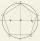Within a regular pentagon ABCDE point P is such that the triangle is equilateral ABP. How big is the angle BCP? Make a sketch.
8. Square gridSquare grid consists of a square with sides of length 1 cm. Draw in it at least three different patterns such that each had a content of 6 cm2 and circumference 12 cm and that their sides is in square grid.
9. MO - trianglesOn the AB and AC sides of the triangle ABC lies successive points E and F, on segment EF lie point D. The EF and BC lines are parallel and is true this ratio FD:DE = AE:EB = 2:1. The area of ABC triangle is 27 hectares and line segments EF, AD, and DB se
10. Amazing numberAn amazing number is name for such even number, the decomposition product of prime numbers has exactly three not necessarily different factors and the sum of all its divisors is equal to twice that number. Find all amazing numbers.
11. Lord Ram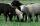When lord Ram founded the breed white sheep was 8 more than black. Currently white sheep are four times higher than at the beginning and black three times more than at the beginning. White sheep is now 42 more than the black. How many white and black sh
12. MO circlesJuro built the ABCD square with a 12 cm side. In this square, he scattered a quarter circle that had a center at point B passing through point A and a semicircle l that had a center at the center of the BC side and passed point B. He would still build a ci
13. Trapezoid MO-5-Z8ABCD is a trapezoid that lime segment CE divided into a triangle and parallelogram as shown. Point F is the midpoint of CE, DF line passes through the center of the segment BE and the area of the triangle CDE is 3 cm2. Determine the area of the trapezoid A
14. Tunnels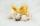Mice had built an underground house consisting of chambers and tunnels: • each tunnel leading from the chamber to the chamber (none is blind) • from each chamber lead just three tunnels into three distinct chambers, • from each chamber mice can get to any
15. Katy MO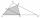Kate draw triangle ABC. Middle of AB have mark as X and the center of the side AC as Y. On the side BC wants to find the point Z such that the content area of a 4gon AXZY was greatest. What part of the triangle ABC can maximally occupy 4-gon AXZY?
16. Eight blocks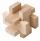Dana had the task to save the eight blocks of these rules: 1. Between two red cubes must be a different color. 2. Between two blue must be two different colors. 3. Between two green must be three different colors. 4. Between two yellow blocks must be four
17. Mrak - cloud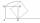It is given segment AB of length 12 cm, where one side of the square MRAK laid on it. MRAK's side length 2 cm shown. MRAK gradually flips along the line segment AB the point R leaves a paper trail. Draw the whole track of point R until square can do the.
18. Three friends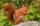Three friends squirrels together went to collect hazelnuts. Zrzecka he found more than twice Pizizubka and Ouska even three times more than Pizizubka. On the way home they talked while eating and was cracking her nuts. Pizizubka eaten half of all nuts whi
19. Cakes Z8-I-5Mom brought 10 cakes of three types: kokosek was less than laskonek and most were caramel cubes. John chose two different kinds of cakes, Stephan did the same and for Margerith leave only the cakes of the same type. How many kokosek, laskonek and caramel c
20. Average ageThe average age of all people at the celebration was equal to the number of people present. After the departure of one person who was 29 years old, average age was again equal to the number present. How many people were originally to celebrate?

Do you have an interesting mathematical example that you can't solve it? Enter it, and we can try to solve it.

To this e-mail address, we will reply solution; solved examples are also published here. Please enter e-mail correctly and check whether you don't have a full mailbox.